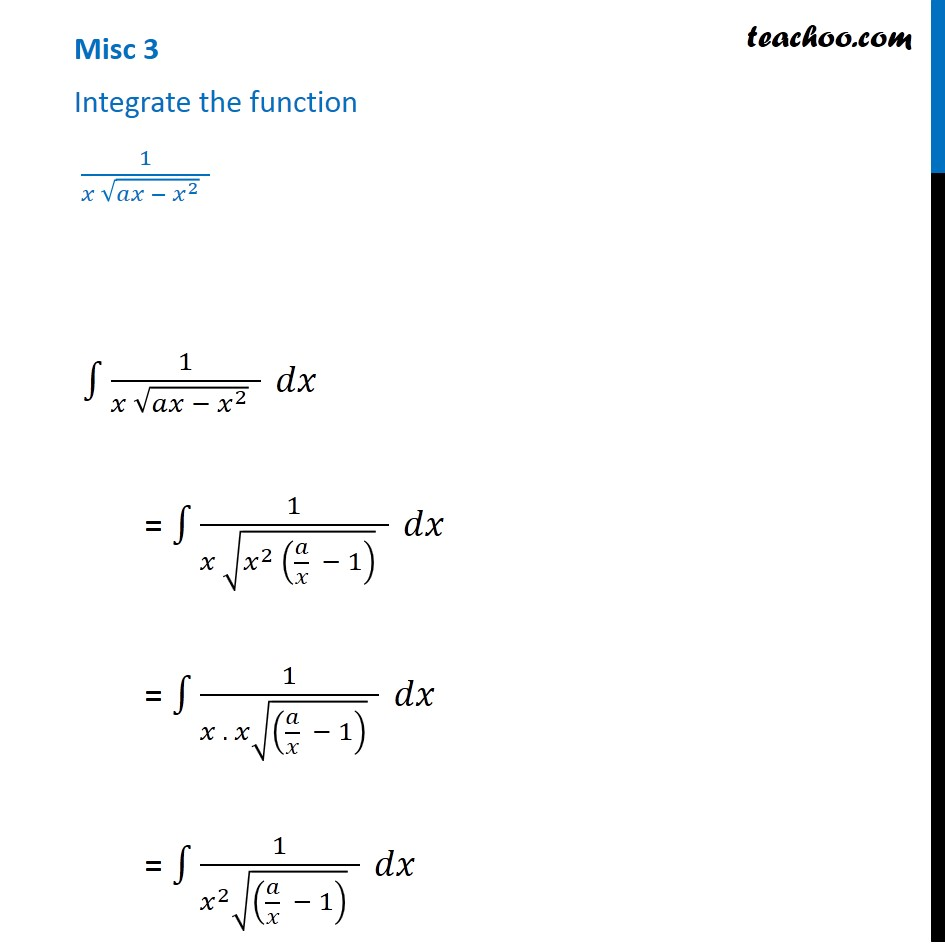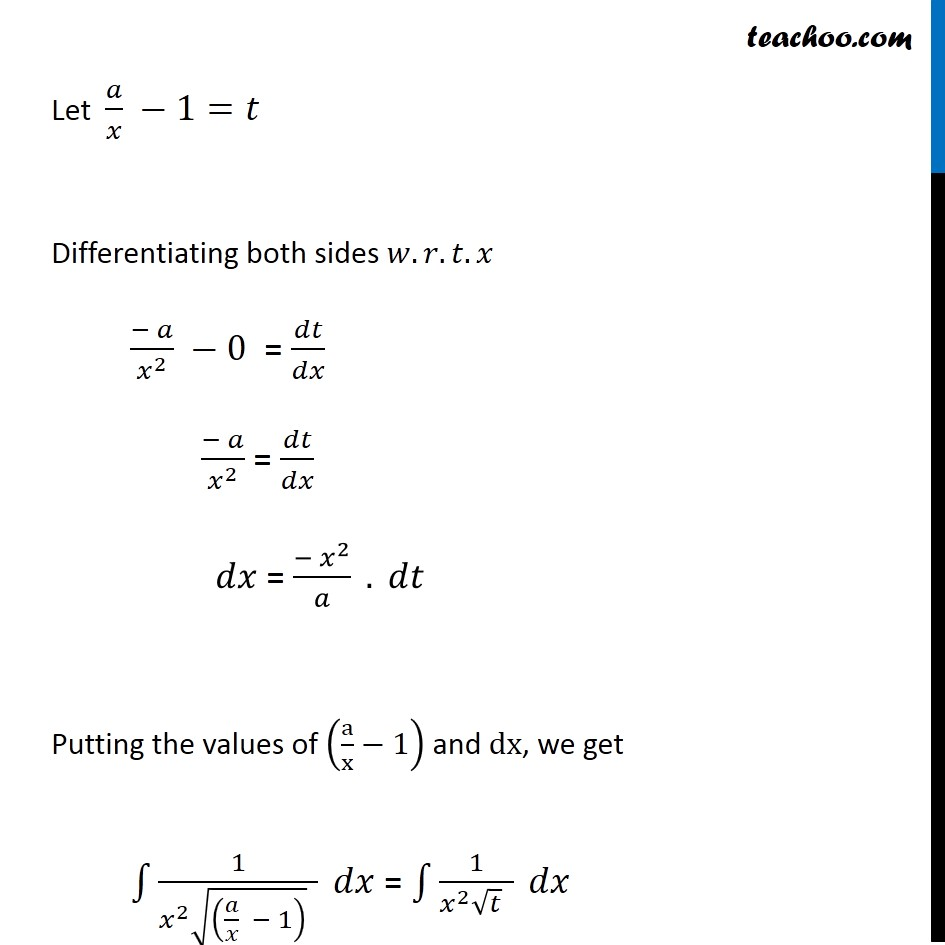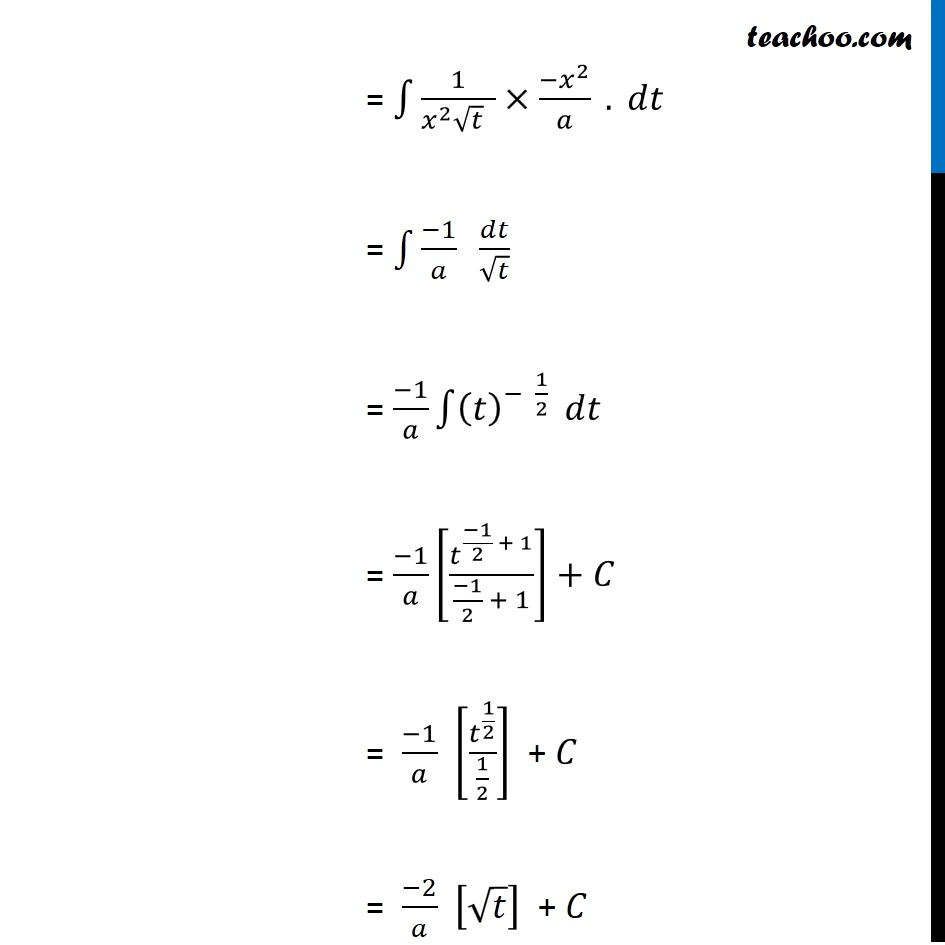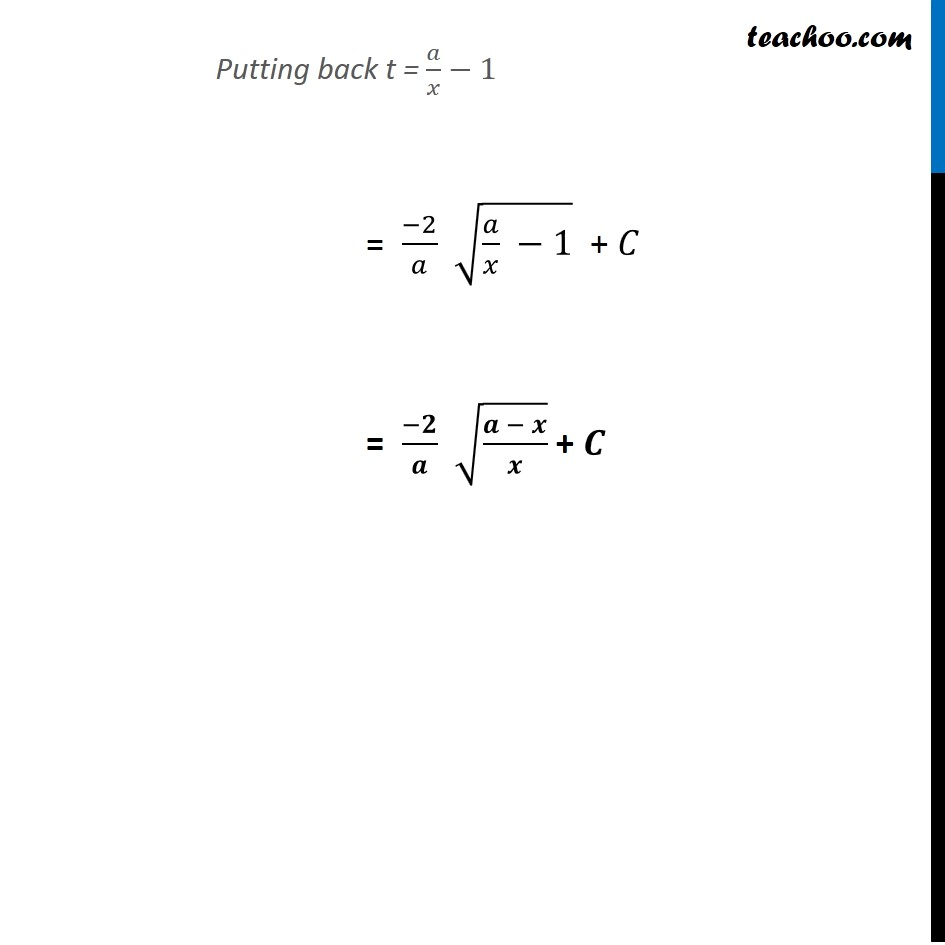Miscellaneous

Chapter 7 Class 12 Integrals
Serial order wiseLearn in your speed, with individual attention - Teachoo Maths 1-on-1 Class

### Transcript

Misc 3 Integrate the function 1/(𝑥 √(𝑎𝑥 − 𝑥^2 ) ) ∫1▒1/(𝑥 √(𝑎𝑥 − 𝑥^2 ) ) 𝑑𝑥 = ∫1▒1/(𝑥 √(𝑥^2 (𝑎/𝑥 − 1) ) ) 𝑑𝑥 = ∫1▒1/(𝑥 . 𝑥√((𝑎/𝑥 − 1) ) ) 𝑑𝑥 = ∫1▒1/(𝑥^2 √((𝑎/𝑥 − 1) ) ) 𝑑𝑥 Let 𝑎/𝑥 −1=𝑡 Differentiating both sides 𝑤.𝑟.𝑡.𝑥 (− 𝑎)/𝑥^2 −0 = 𝑑𝑡/𝑑𝑥 (− 𝑎)/𝑥^2 = 𝑑𝑡/𝑑𝑥 𝑑𝑥 = (− 𝑥^2)/𝑎 . 𝑑𝑡 Putting the values of (a/x−1) and dx, we get ∫1▒1/(𝑥^2 √((𝑎/𝑥 − 1) ) ) 𝑑𝑥 = ∫1▒1/(𝑥^2 √𝑡 ) 𝑑𝑥 = ∫1▒1/(𝑥^2 √𝑡 )×(−𝑥^2)/𝑎 . 𝑑𝑡 = ∫1▒(−1)/𝑎 𝑑𝑡/√𝑡 = (−1)/𝑎 ∫1▒(𝑡)^(− 1/2) 𝑑𝑡 = (−1)/𝑎 [𝑡^((−1)/2 + 1)/((−1)/2 + 1)]+𝐶 = (−1)/𝑎 [𝑡^(1/2)/(1/2)] + 𝐶 = (−2)/𝑎 [√𝑡] + 𝐶 Putting back t = 𝑎/𝑥−1 = (−2)/𝑎 √(𝑎/𝑥 −1) + 𝐶 = (−𝟐)/𝒂 √((𝒂 − 𝒙)/𝒙) + 𝑪